# 高级计算机图形学:曲线建模理论

## Modeling Curves

Posted by R1NG on September 26, 2022 Viewed Times

# 曲线建模理论

## 1. 对曲线的基本定义

1. 一系列的点坐标组成的一维数组
2. 一个 (一维或 $n$ 维的, 取决于曲线所在空间维度的) 映射.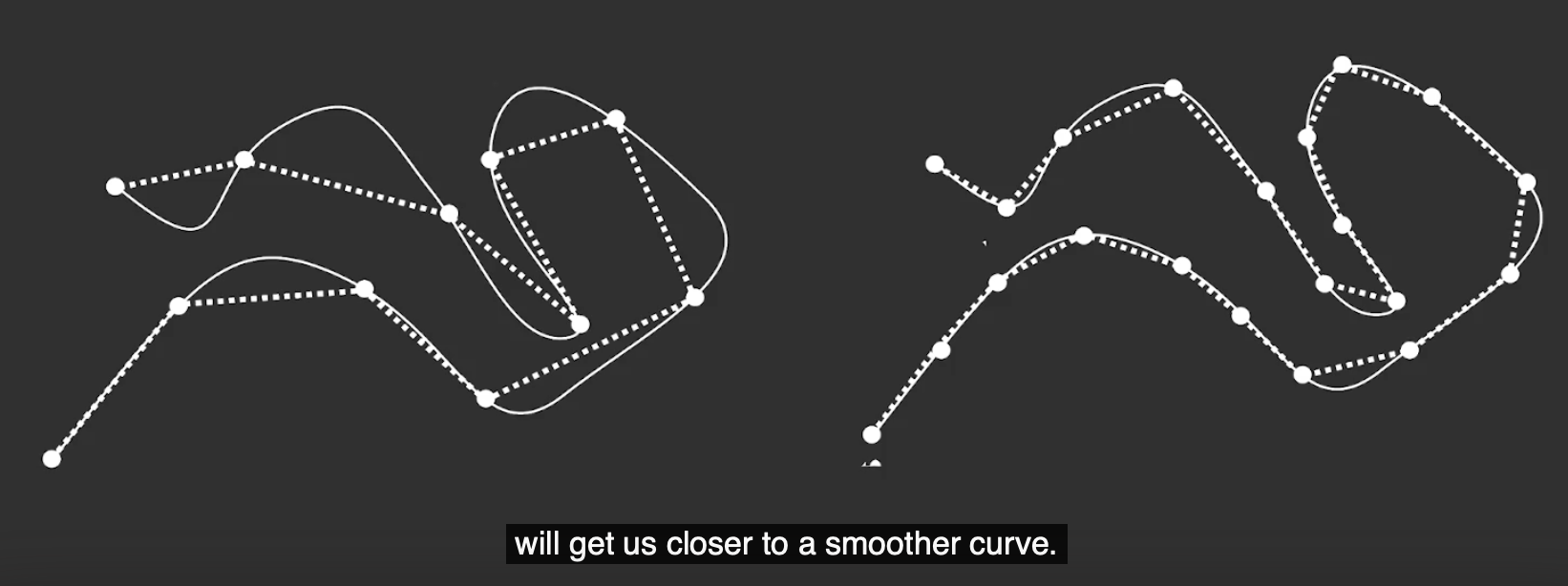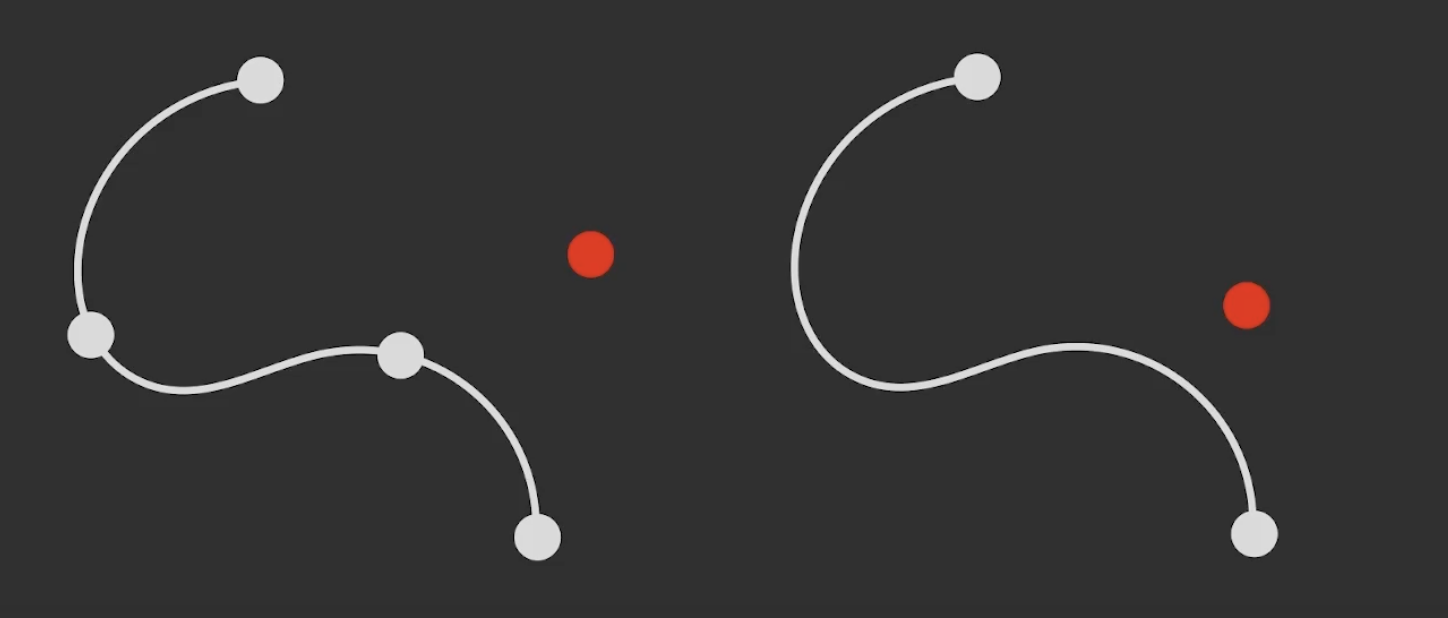## 2. 三次贝塞尔曲线

1. 给定四个控制点: 两个插值点 $P_0, P_3$ 和两个近似点 $P_1, P_2$.
2. 对两个插值点进行曲线插值, 此时该曲线同时可视为对另外两个点的近似.

$P_0$, $P_1$, $P_2$, $P_3$ 四个点在平面或在三维空间中定义了三次贝塞尔曲线.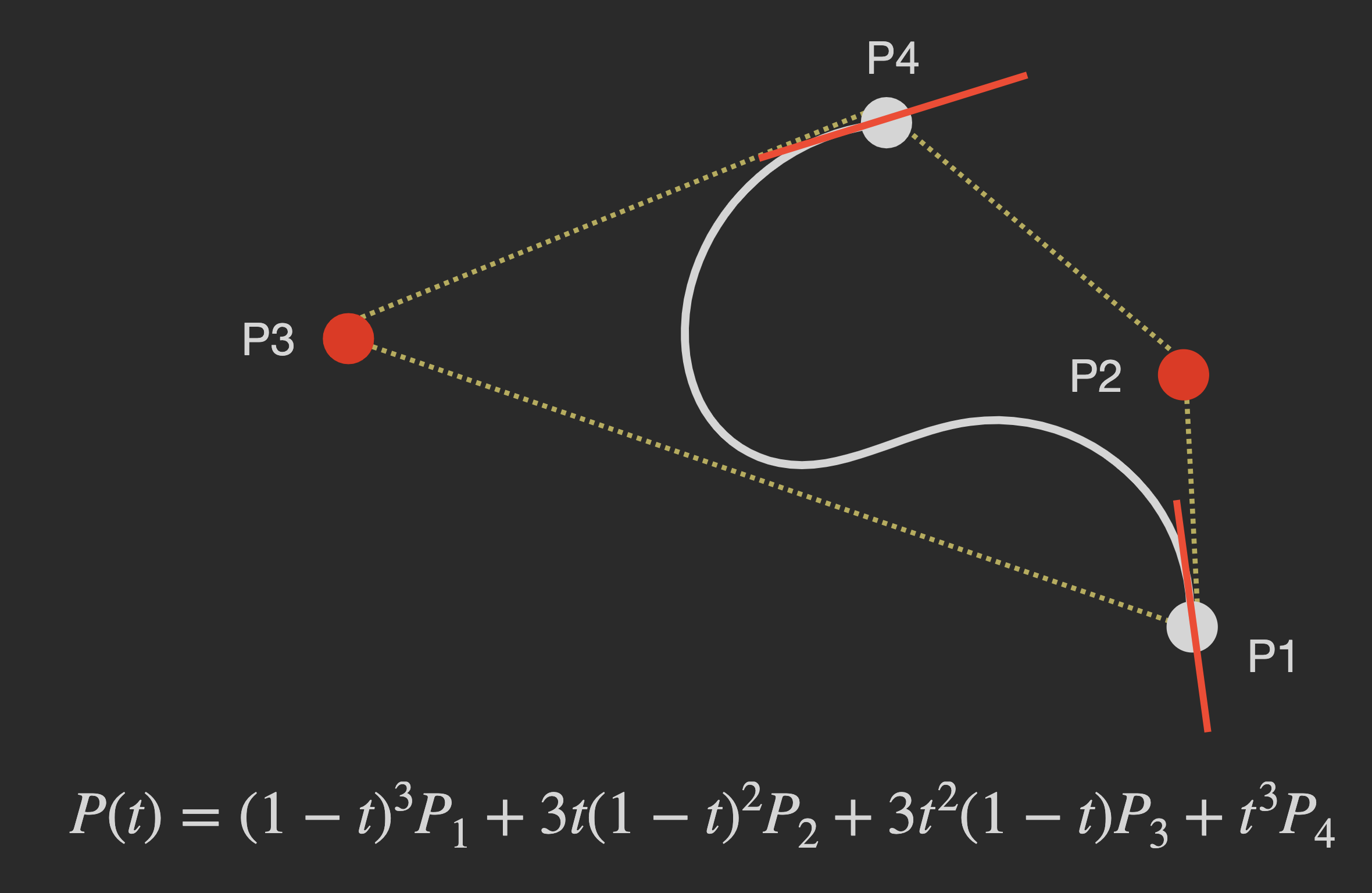$P(t) = P_0(1-t)^3 + 3P_1t(1-t)^2 + 3P_2t^2(1-t) + P_3t^3.$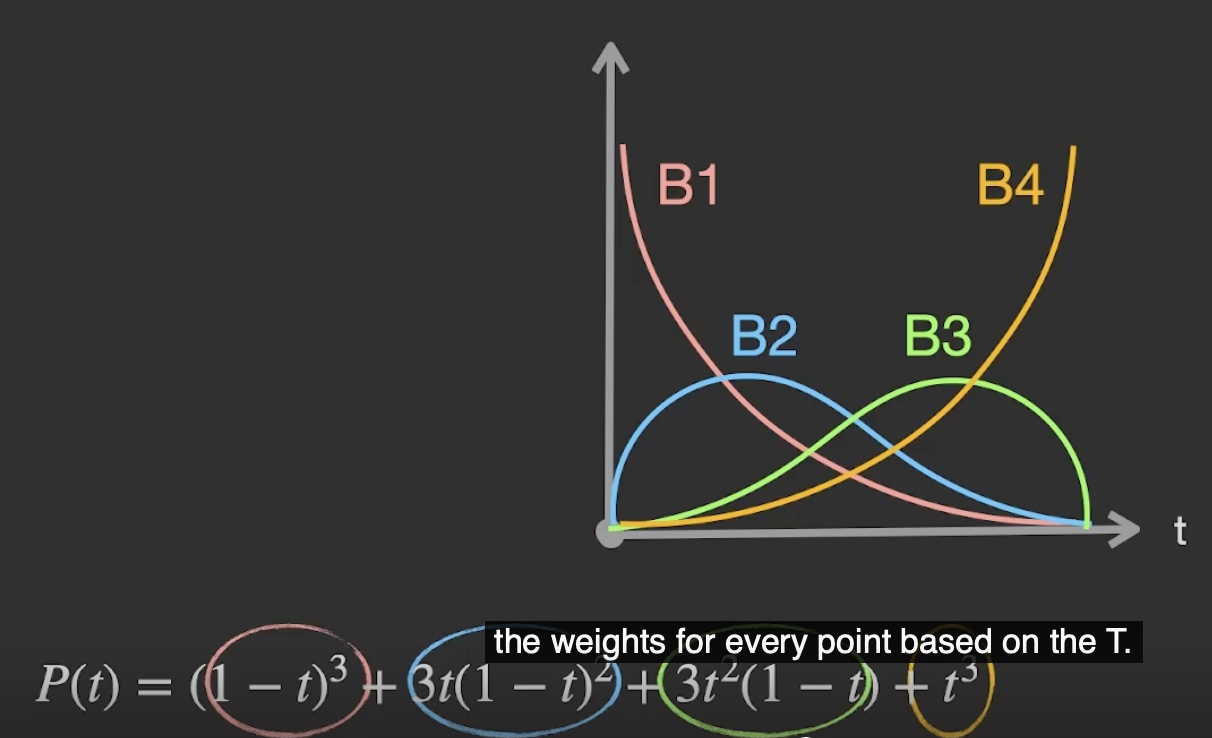### 2.1 三次贝塞尔曲线的矩阵运算表示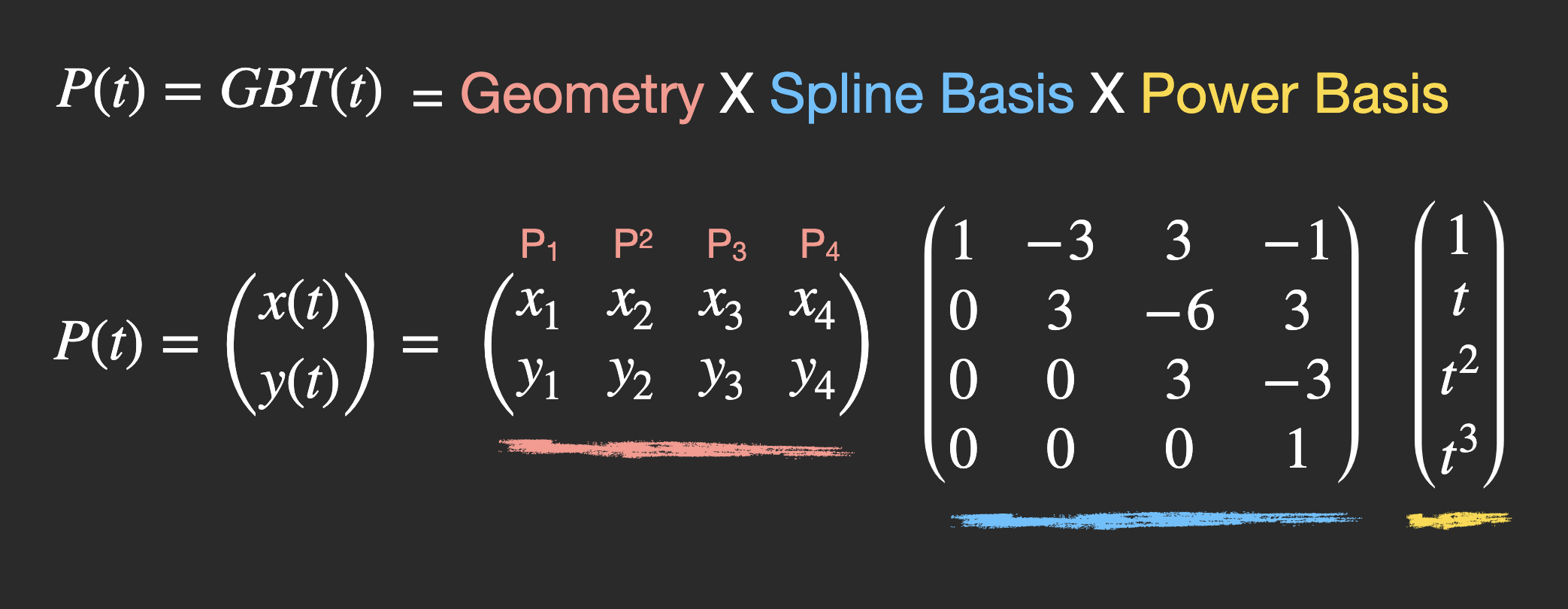### 2.2 伯恩斯坦基

$B_{i, n}(t) = \binom{n}{i} t^i (1-t)^{n-i}.$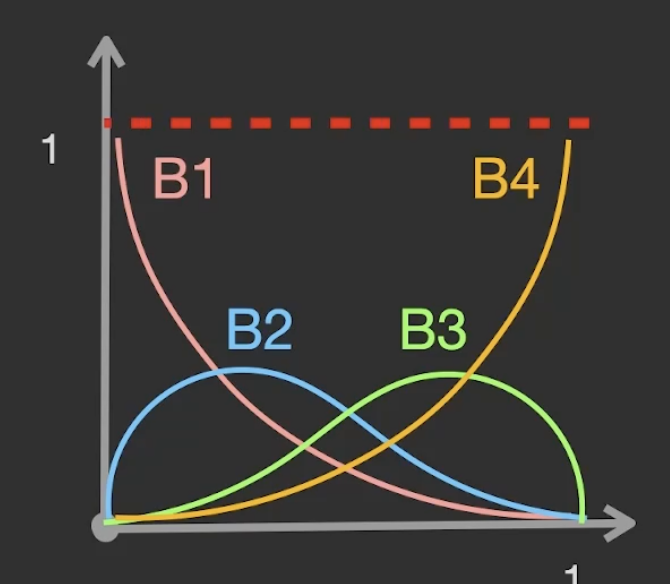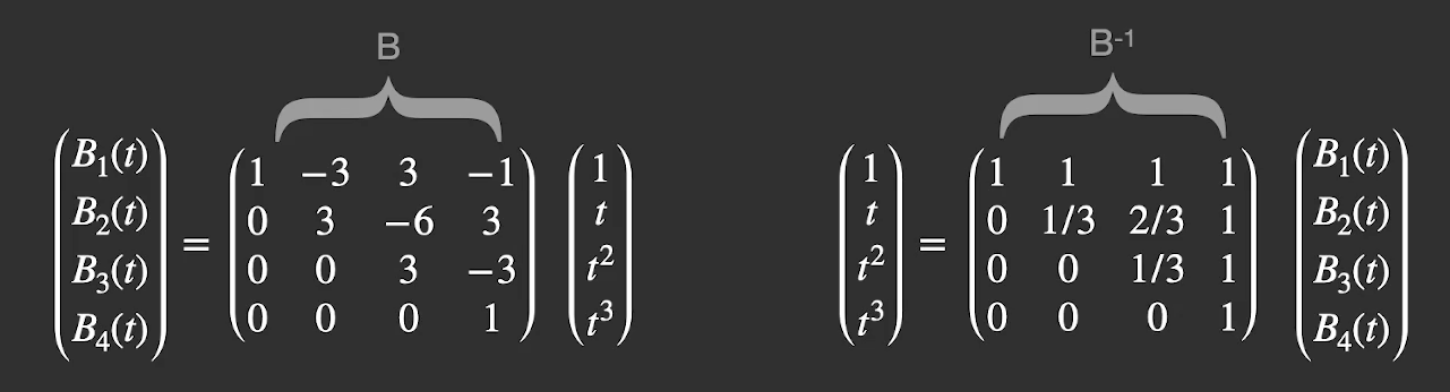### 2.3 升阶: 用高维贝塞尔曲线建模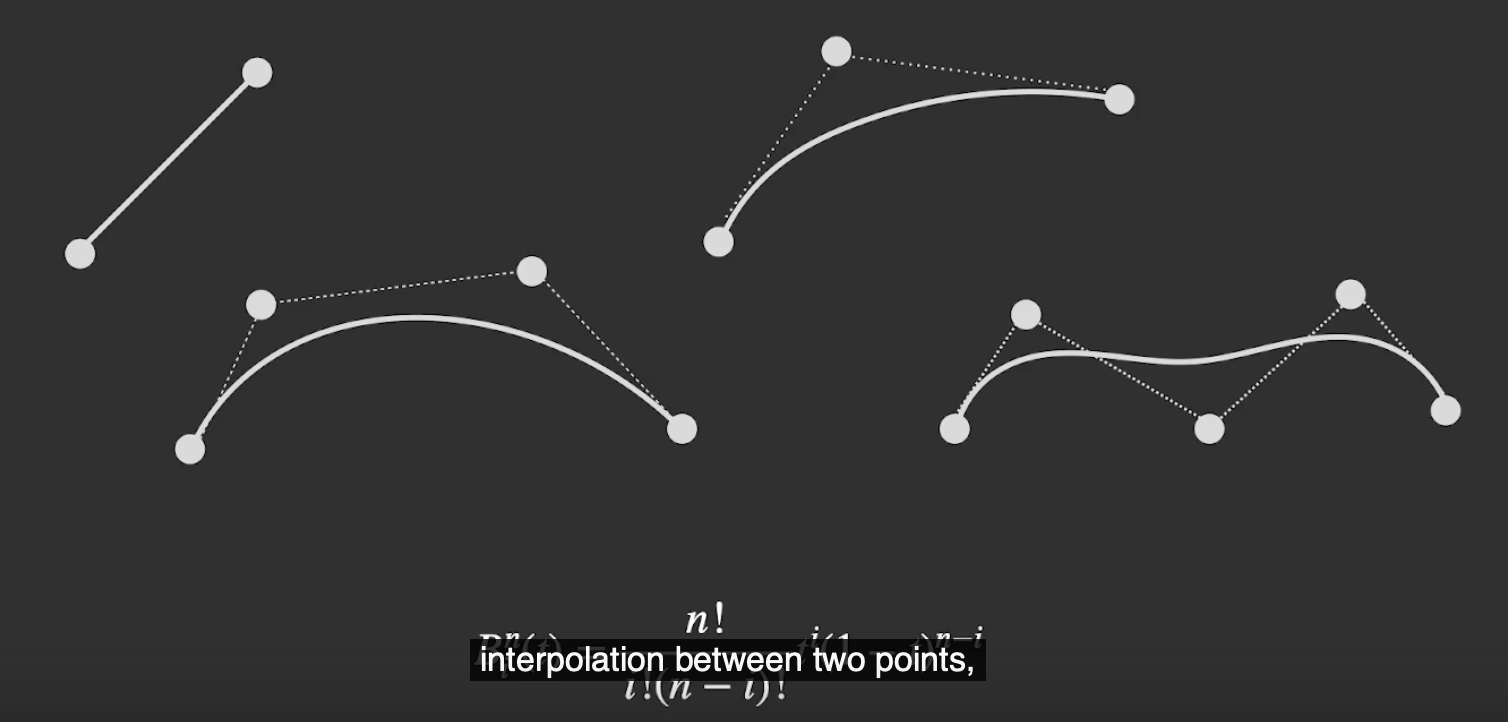### 2.4 简化: 贝塞尔曲线的拆分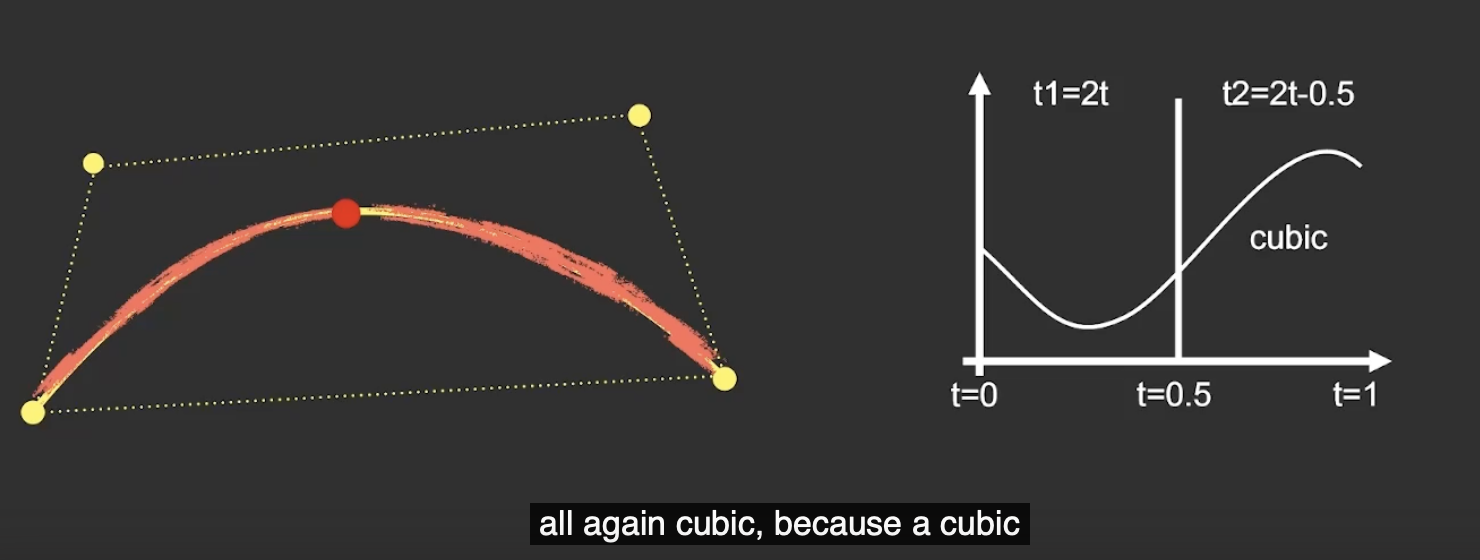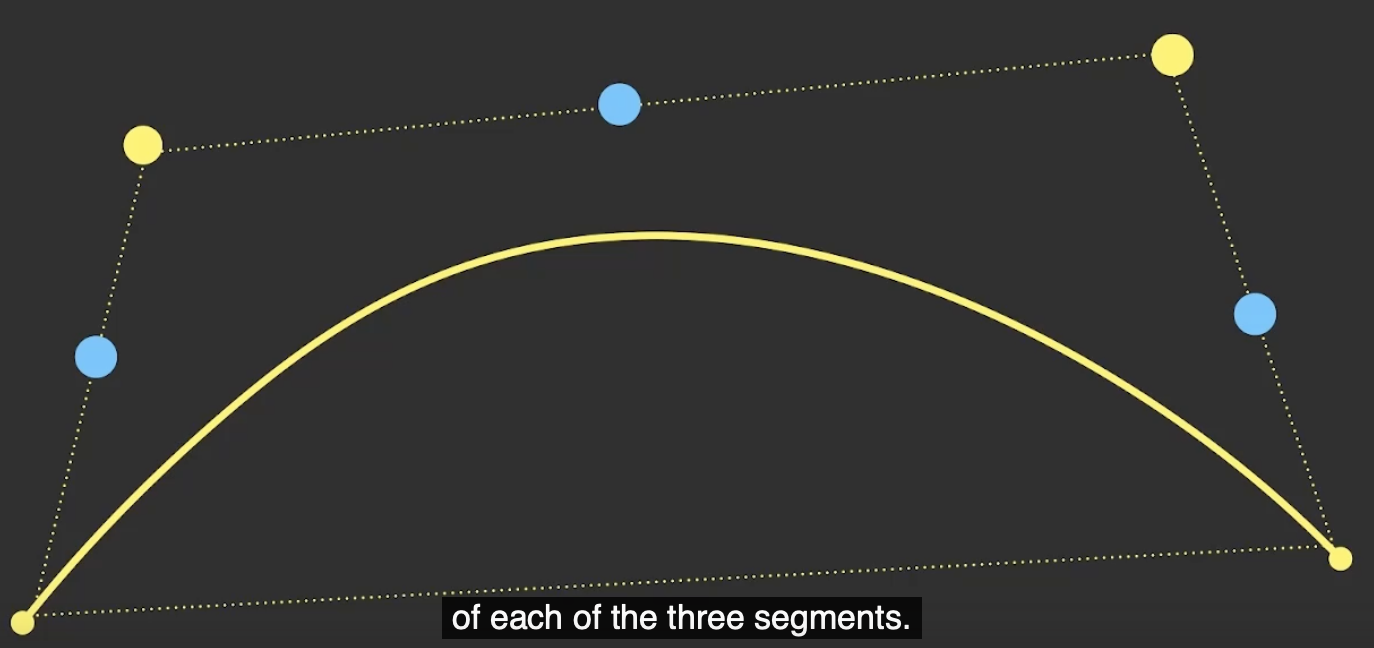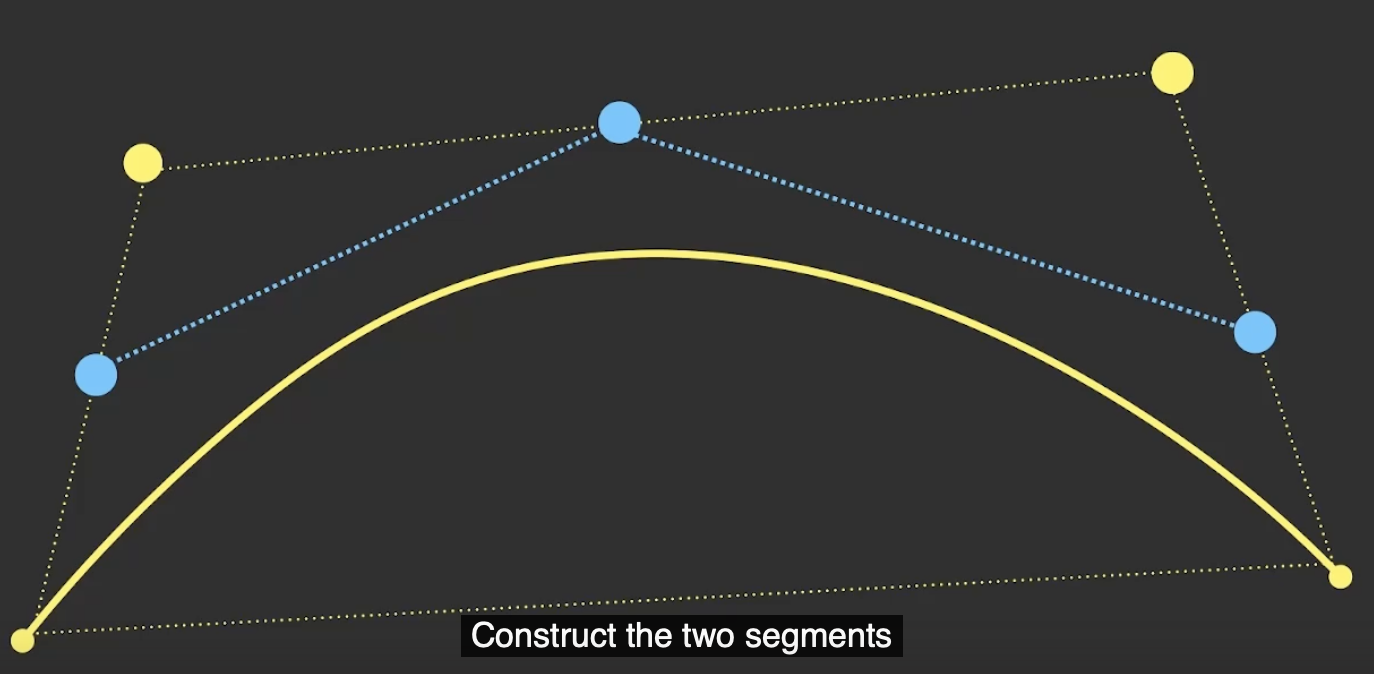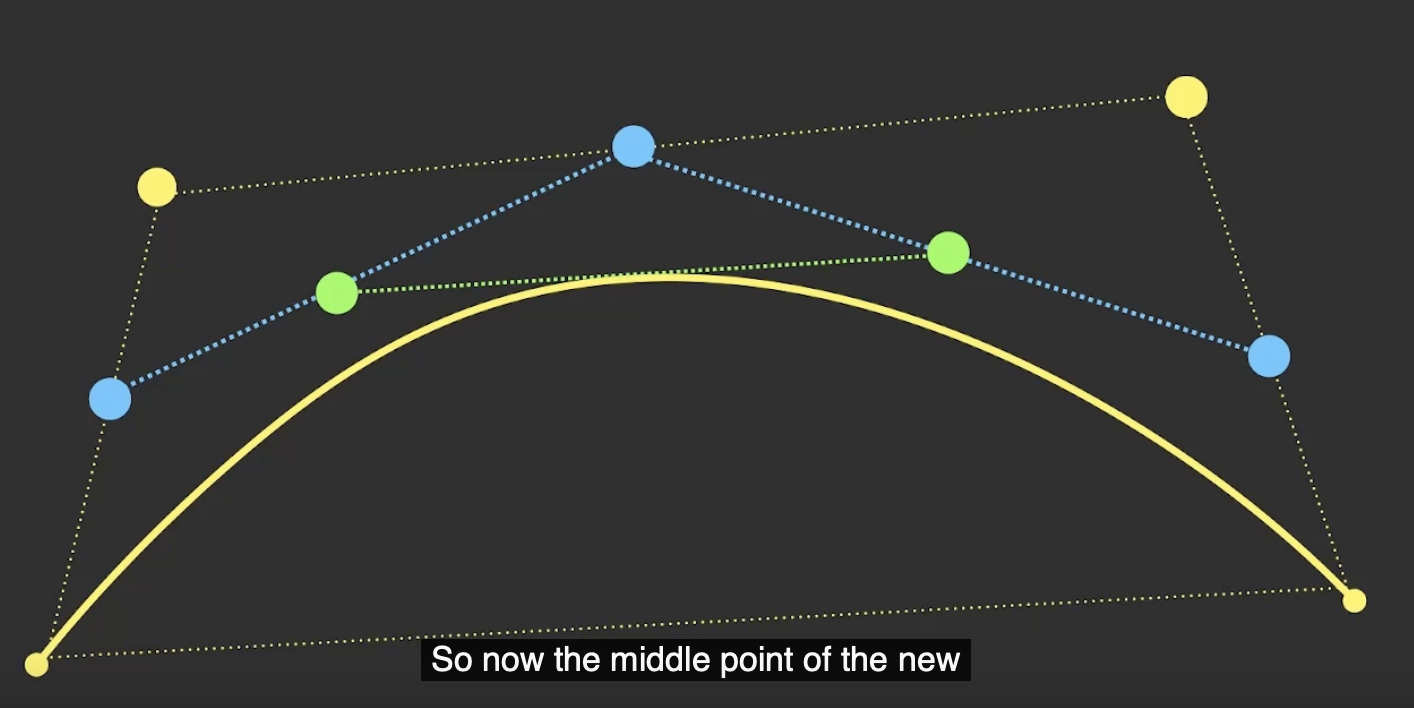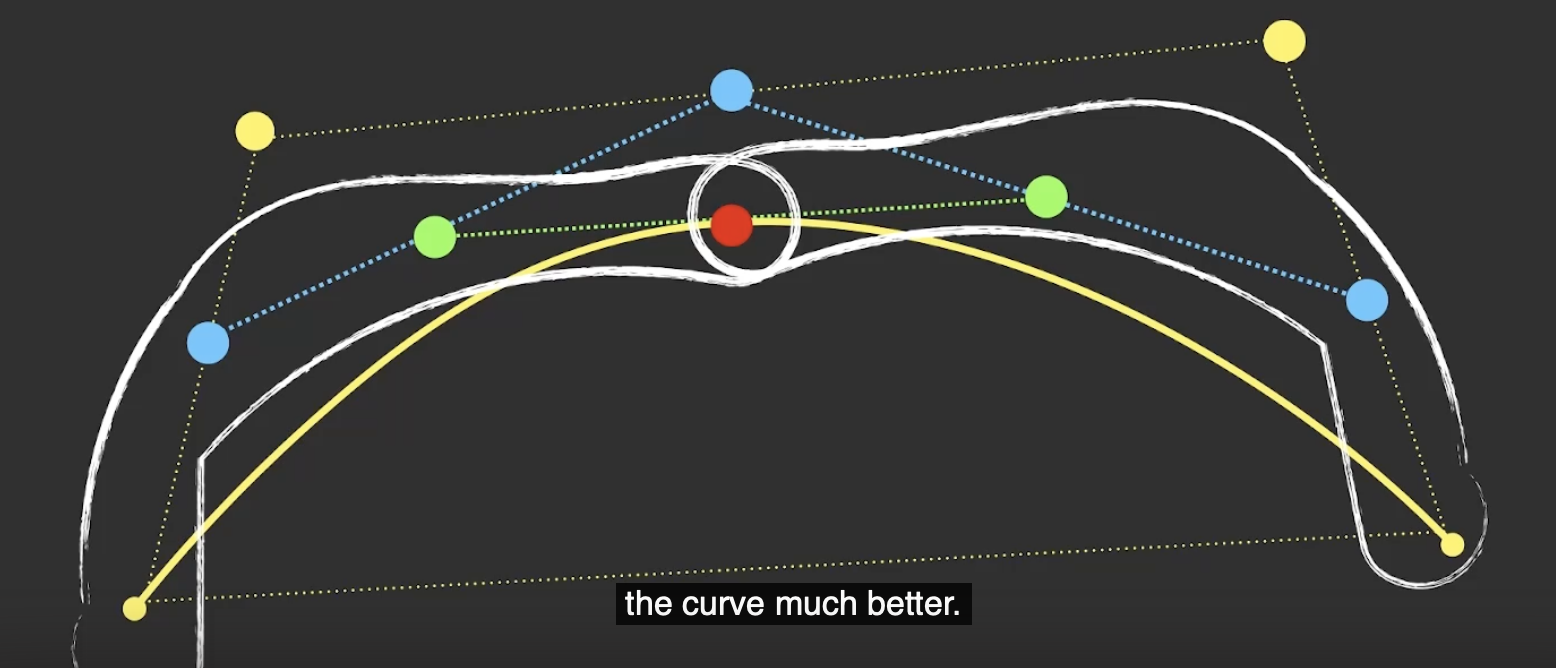## 3. 曲线的微分性质 (Differential Property)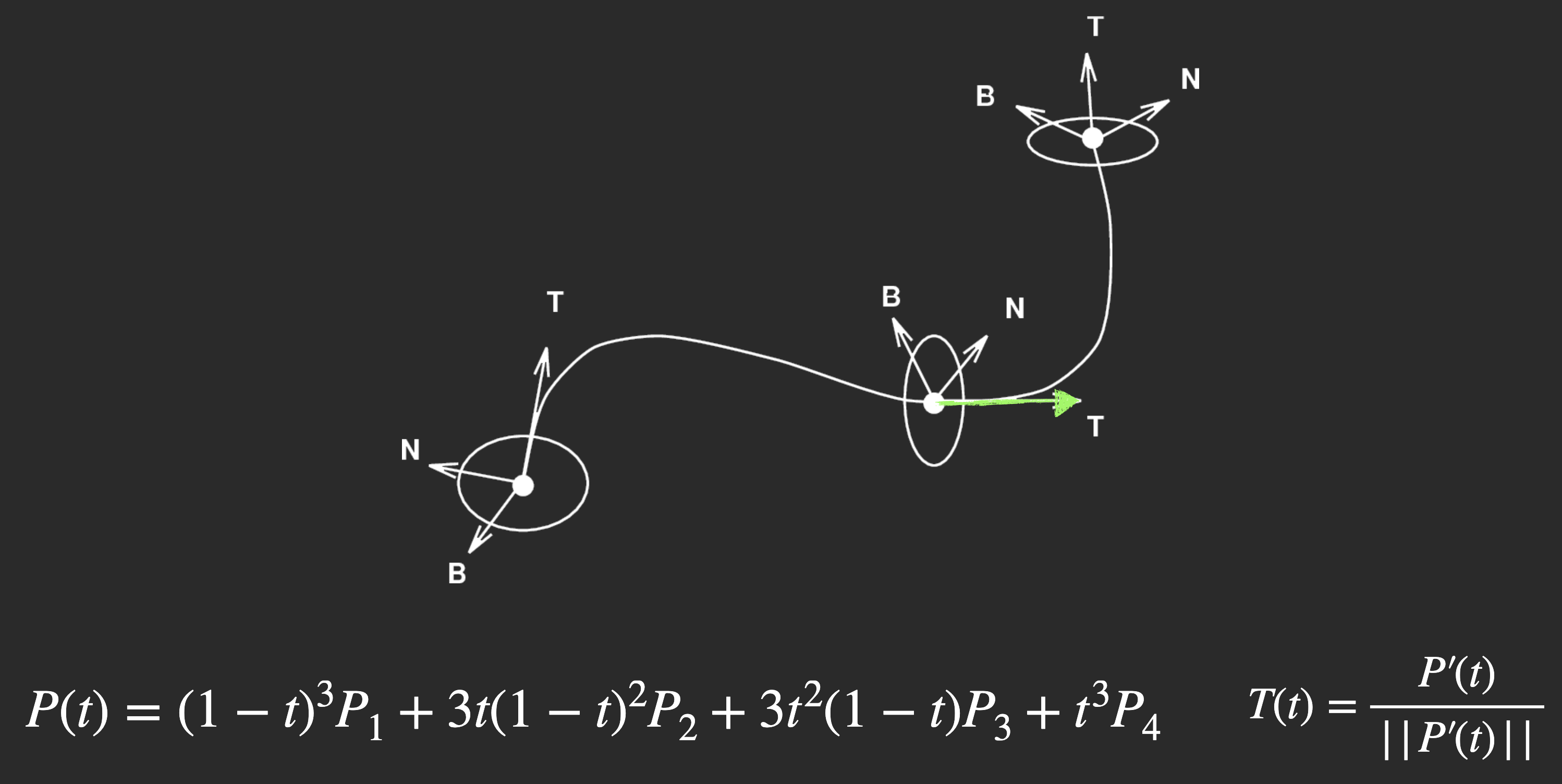1. $C0$: 最基本的 连续性指标, 只要两条曲线 相连 即可, 不关心它们之间的连接平滑与否.

2. $G1$: 又称为 几何连续性 (Geometry Continuity), 只要两条曲线在它们相接的地方 切向量的方向一致 即可满足. 在大多数情况下 (如建模) 曲线连接只要满足 $G1$ 性质即可.

3. $C1$: 称为 参数连续性 (Parametric Continuity), 两条曲线需要在 连接处拥有相同的切向量 (也就是一阶导数相同, 切向量的方向和大小都一致, 反映出来的信息就是: 如果这条曲线是某个物体的运动曲线, 则物体在这一点上, 运动的 方向速度 都一致). 因此对 动画 而言, $C1$ 级别的曲线连续性已基本足够.

$C2\backslash C2+$: 更强的连续性性质, 在本课程中不详细介绍. 简单来说 $C2$ 需要曲线在连接处 除了切向量相同, 二阶导数也需相同.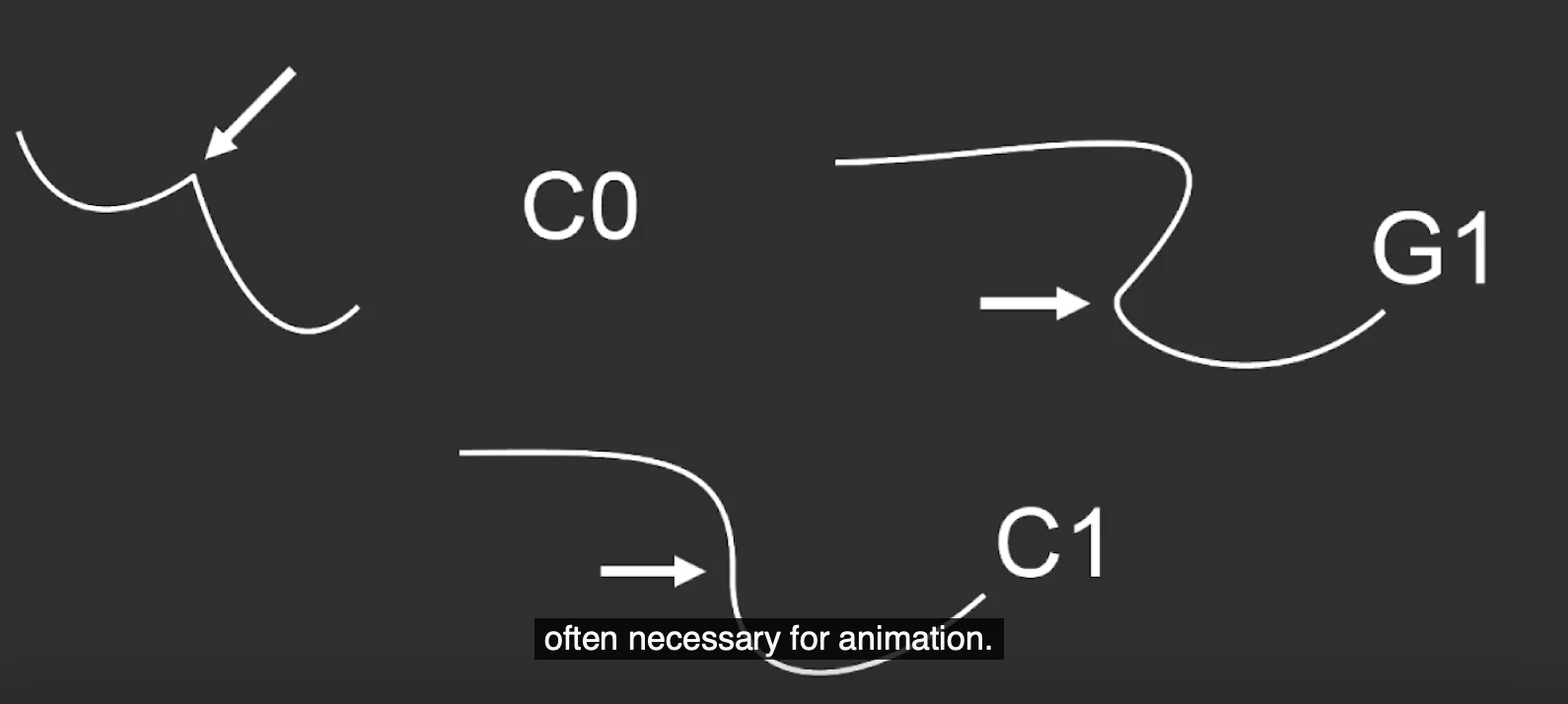## 4. B-样条曲线 (B-Spline)

1. 贝塞尔曲线的 拼接非常复杂 , 要想得到理想的结果需要确保拼接的曲线满足几何连续性或参数连续性.
2. 贝塞尔曲线的次数由其 特征多边形的顶点数 决定 (注意到任何贝塞尔曲线的次数总等于其特征多边形的顶点数 $-1$).
3. 贝塞尔曲线的控制点对曲线形状的影响是 全局 的: 修改任何一个控制点, 整条曲线的形状都会发生变化, 无法对贝塞尔曲线进行 局部修改.

$S: [0, 1] \rightarrow \mathbb{R}^{2}$

$S(t) = \sum_{i=0}^{m}P_{i} b_{i, n}(t), ~~ t \in [0, 1].$

B-样条曲线的主要优点在于:

1. 天然满足最强的 $C2$ 连续性, 无需像贝塞尔曲线那样在拼接时还得检查两条曲线端点处的切线, 确定曲线之间的接合处满足几何连续性或参数连续性, 任意多条B-样条曲线首尾相连得到的曲线必然是 处处满足 $C2$ 连续性 的.
2. 控制点的数量和曲线表达式的次数无关, 由此大大简化了复杂曲线的表达方式.
3. 调整某个控制点只会造成 曲线局部的变化 而不会导致贝塞尔曲线 “牵一发而动全身” 的效果, 方便在对曲线建模时修改和编辑.

1. 和贝塞尔曲线不同, B-样条曲线 不被要求必须通过任何一个控制点.

2. 和贝塞尔曲线相同, B-样条曲线同样受由控制点围成的区域 (也就是 凸包, Convex Hull) 的限制, 不能超出这个区域.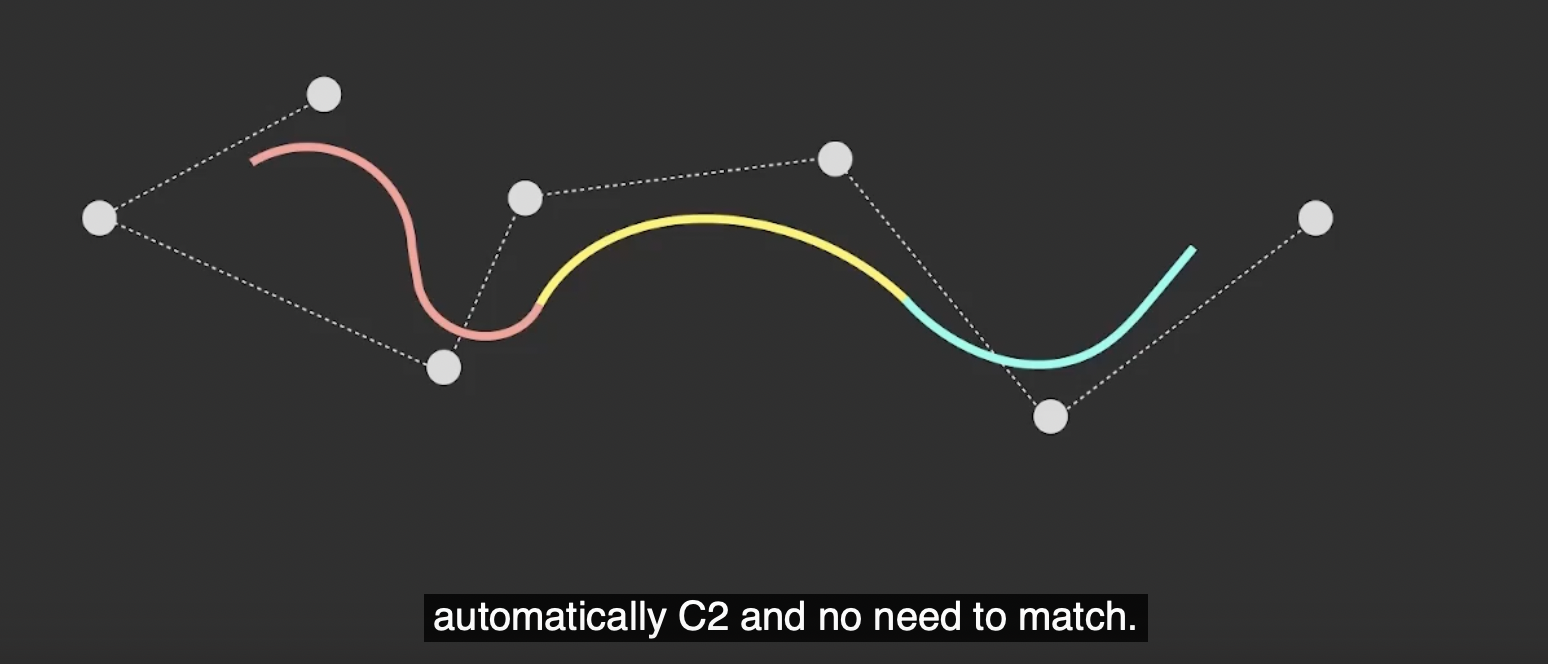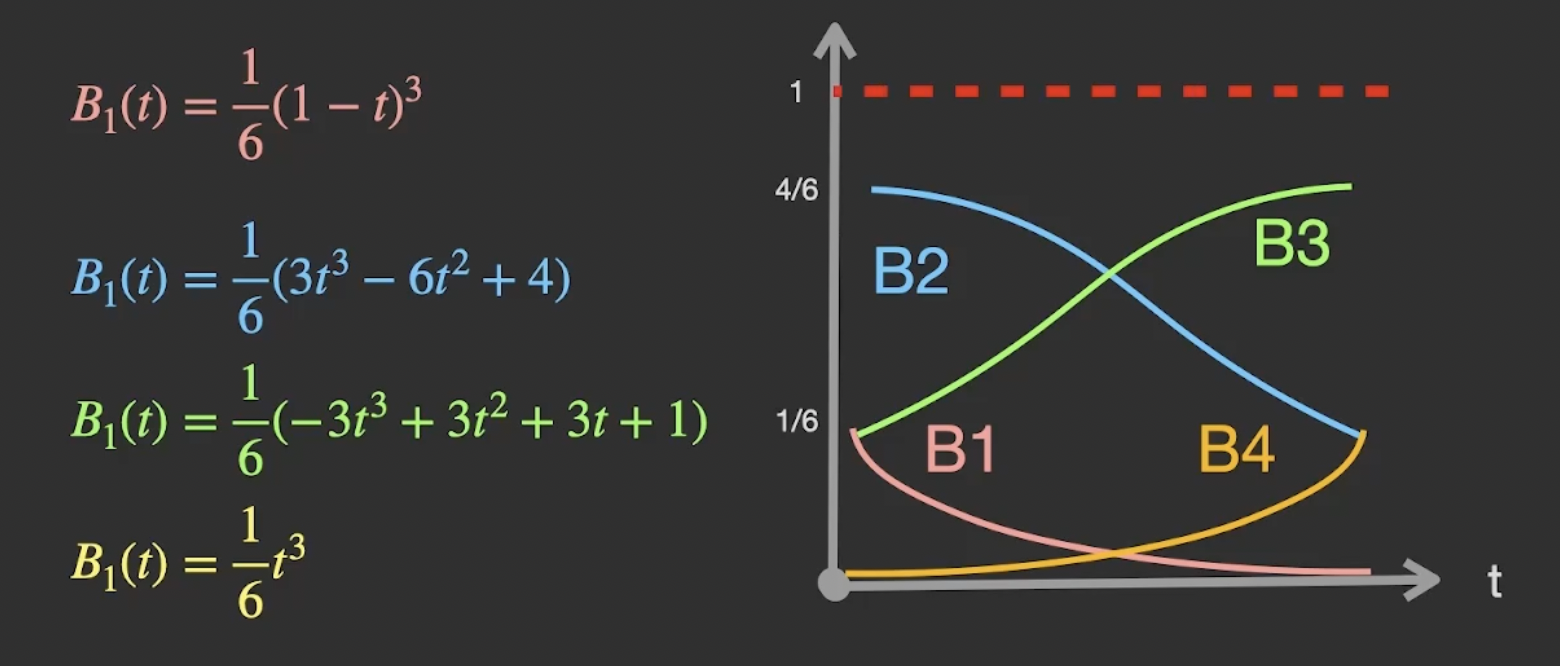### 4.1 B-样条曲线表示和贝塞尔曲线表示的互相转换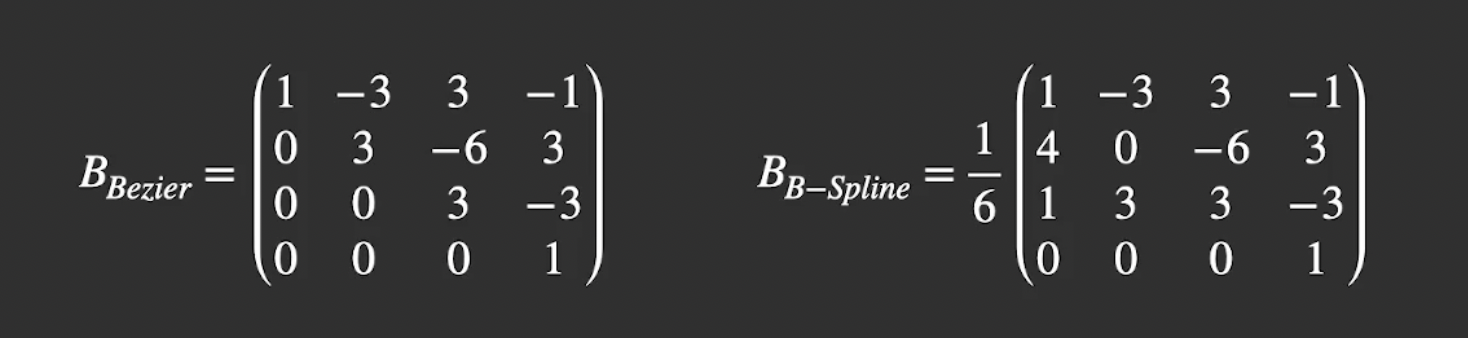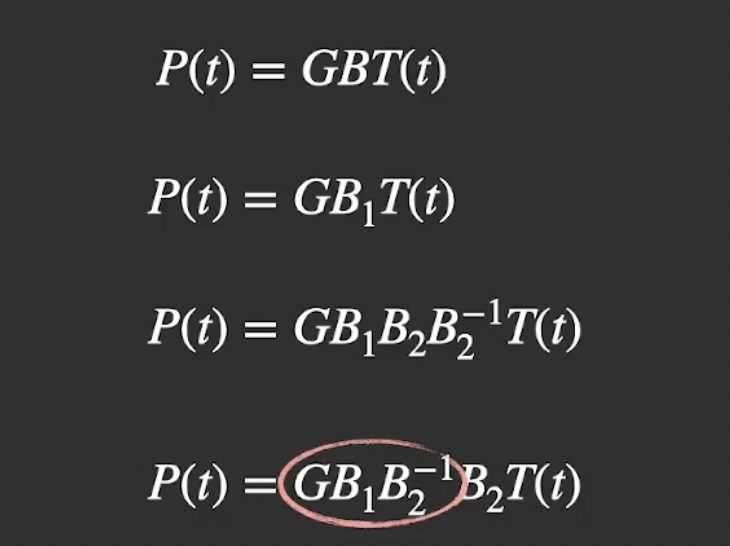$P(t) = GB_2T(t)$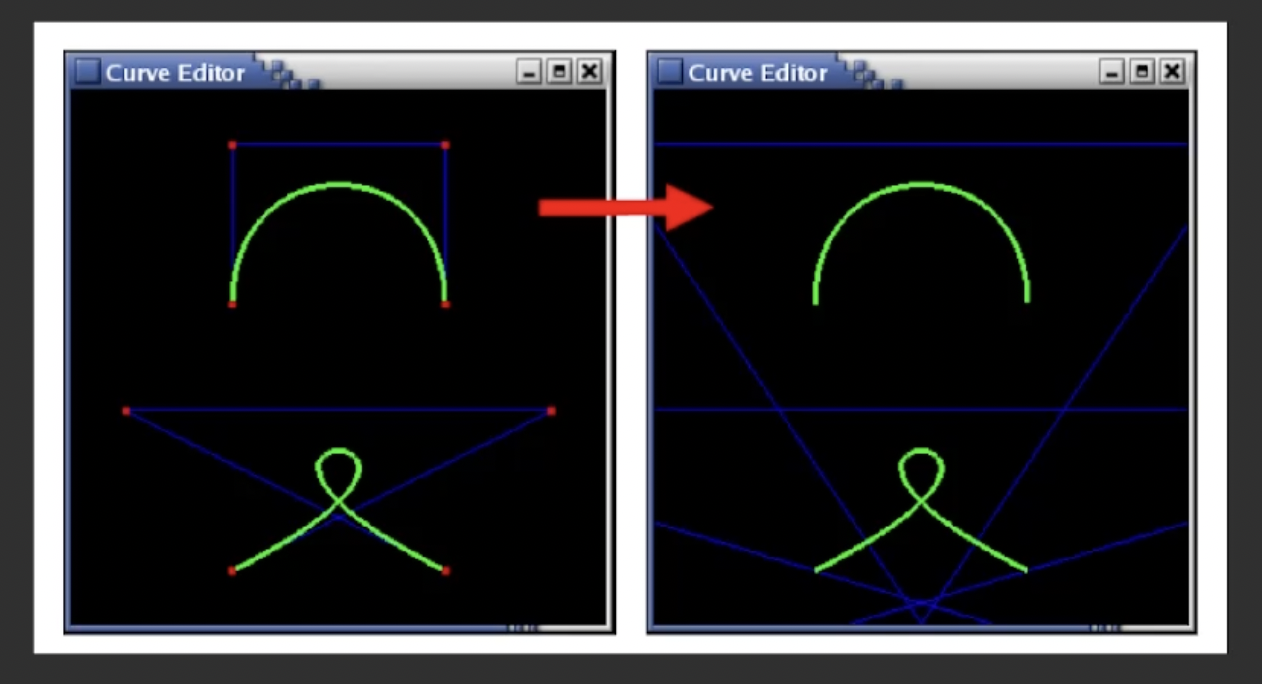### 4.2 非均匀有理样条 NURBS (Non-Uniform Rational B-Spline)